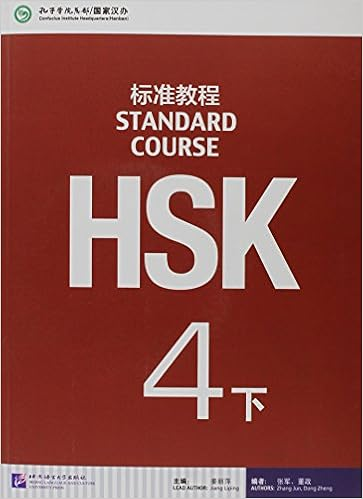Difference between revisions of "Reference:HSK Standard Course 5下"HSK Standard Course 5下, by Liu Chang and Lu Jiang, contains 10 lessons. Authorized by Hanban, HSK Standard Course is developed under the joint efforts of Beijing Language and Culture University Press and Chinese Testing International (CTI). With HSK test papers as its primary source, HSK Standard Course is characterized by a humorous style, familiar topics and a scientific course design.

1. Lesson 19
2. Lesson 20
3. Lesson 21
4. Lesson 22
5. Lesson 23
6. Lesson 24
7. Lesson 25
8. Lesson 26
9. Lesson 27
10. Lesson 28
11. Lesson 29
12. Lesson 30
13. Lesson 31
14. Lesson 32
15. Lesson 33
16. Lesson 34
17. Lesson 35
18. Lesson 36